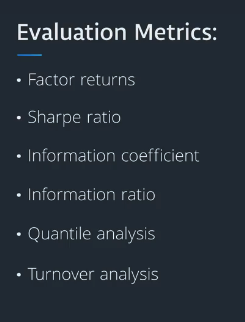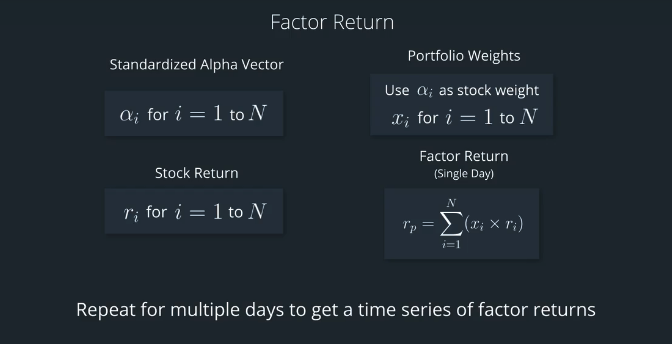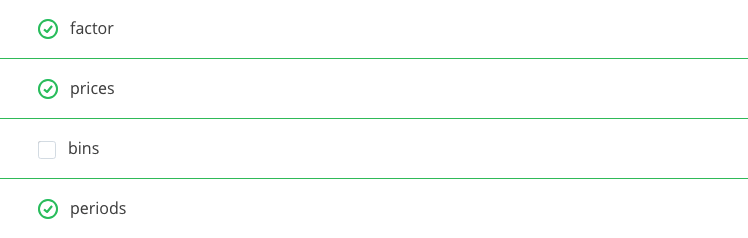# AI For Trading:Factor Returns (67)

## Factor returns

Once we've calculated an Alpha factor, we can calculate some evalution metrics that let us compare it to other Alpha factors and get a sense for how it might perform when used to design a real-world portfolio.

These metrics include factor returns, Sharpe retio and so on.We evaluate Alpha factors to help us decide whether we'll use them in creating a combined Alpha factor, which we'll then use within portfolio optimization.

One useful construct, is the return of the factor, which is called the factor return. The idea is that this is the return that a theoretical portfolio designed to capitalize on the arbitrage idea presented by the factor would produce.

You can think of this as a way to directly measure the returns,your portfolio would have if their weights were determined purely by the Alpha factor.

To calculate the factor return we create a theoretical portfolio in which the weights for each stock on each day are set equal to the Alpha value for each stock on that day.

So, every day we use the prior day's known data to calculate an Alpha vector,which we would standardize to have mean zero,and the sum of absolute values equal to one.## Factor returns quiz

Forward returns
For this quiz, note that forward return refers to a return that is in the future, or “forward” in time...compared to when the alpha value was calculated. So, if the alpha values are calculated before time “t”, then the forward asset return is calculated with data that occurs later, from time t to time t plus one. We'll discuss this in a video in a little bit later in this lesson.

Looking at the source code for the alphalens: factor_returns

The alphalens.performance.factor_returns function looks like this:

 weights = factor_weights(factor_data, demeaned, group_adjust, equal_weight)
weighted_returns = factor_data[utils.get_forward_returns_columns(factor_data.columns)] \
.multiply(weights, axis=0)

if by_asset:
returns = weighted_returns
else:
returns = weighted_returns.groupby(level='date').sum()

# preserve freq, which contains trading calendar information
returns.index.freq = factor_data.index.levels.freq
return returns

What two pieces of information is it multiplying together to get the factor return for each stock?## get_clean_factor_and_forward_returns

Looking at the documentation for the alphalens function get_clean_factor_and_forward_returns,

This function formats factor and pricing data, and also chooses the frequency of the formatted data. So for instance, given daily data, we could output the formatted data to be weekly.

Which three parameters do you think we would set in order to call this get_clean_factor_and_forward_returns function so that we could get formatted data for factor values, forward returns, and at a desired frequency (for example, 5 trading days for weekly frequency)?## Install packages

import sys
!{sys.executable} -m pip install -r requirements.txt
import cvxpy as cvx
import numpy as np
import pandas as pd
import time
import os
import quiz_helper
import matplotlib.pyplot as plt
%matplotlib inline
plt.style.use('ggplot')
plt.rcParams['figure.figsize'] = (14, 8)

### data bundle

import os
import quiz_helper
from zipline.data import bundles
os.environ['ZIPLINE_ROOT'] = os.path.join(os.getcwd(), '..', '..','data','module_4_quizzes_eod')
ingest_func = bundles.csvdir.csvdir_equities(['daily'], quiz_helper.EOD_BUNDLE_NAME)
bundles.register(quiz_helper.EOD_BUNDLE_NAME, ingest_func)
print('Data Registered')

### Build pipeline engine

from zipline.pipeline import Pipeline
from zipline.pipeline.factors import AverageDollarVolume
from zipline.utils.calendars import get_calendar

universe = AverageDollarVolume(window_length=120).top(500)
engine = quiz_helper.build_pipeline_engine(bundle_data, trading_calendar)

### View Data

With the pipeline engine built, let's get the stocks at the end of the period in the universe we're using. We'll use these tickers to generate the returns data for the our risk model.

universe_end_date = pd.Timestamp('2016-01-05', tz='UTC')

universe_tickers = engine\
.run_pipeline(
Pipeline(screen=universe),
universe_end_date,
universe_end_date)\
.index.get_level_values(1)\
.values.tolist()

universe_tickers

Get Returns data

from zipline.data.data_portal import DataPortal

data_portal = DataPortal(
bundle_data.asset_finder,
adjustment_reader=bundle_data.adjustment_reader)

## Get pricing data helper function

def get_pricing(data_portal, trading_calendar, assets, start_date, end_date, field='close'):
end_dt = pd.Timestamp(end_date.strftime('%Y-%m-%d'), tz='UTC', offset='C')
start_dt = pd.Timestamp(start_date.strftime('%Y-%m-%d'), tz='UTC', offset='C')

return data_portal.get_history_window(
assets=assets,
end_dt=end_dt,
bar_count=end_loc - start_loc,
frequency='1d',
field=field,
data_frequency='daily')

## get pricing data into a dataframe

returns_df = \
get_pricing(
data_portal,
universe_tickers,
universe_end_date - pd.DateOffset(years=5),
universe_end_date)\
.pct_change()[1:].fillna(0) #convert prices into returns

returns_df

## Sector data helper function

We'll create an object for you, which defines a sector for each stock. The sectors are represented by integers. We inherit from the Classifier class. Documentation for Classifier, and the source code for Classifier

from zipline.pipeline.classifiers import Classifier
from zipline.utils.numpy_utils import int64_dtype
class Sector(Classifier):
dtype = int64_dtype
window_length = 0
inputs = ()
missing_value = -1

def __init__(self):

def _compute(self, arrays, dates, assets, mask):
return np.where(
self.data[assets],
self.missing_value,
)
sector = Sector()

## We'll use 2 years of data to calculate the factor

Note: Going back 2 years falls on a day when the market is closed. Pipeline package doesn't handle start or end dates that don't fall on days when the market is open. To fix this, we went back 2 extra days to fall on the next day when the market is open.

factor_start_date = universe_end_date - pd.DateOffset(years=2, days=2)
factor_start_date

## Create smoothed momentum factor

from zipline.pipeline.factors import Returns
from zipline.pipeline.factors import SimpleMovingAverage

# create a pipeline called p
p = Pipeline(screen=universe)
# create a factor of one year returns, deman by sector, then rank
factor = (
demean(groupby=Sector()). #we use the custom Sector class that we reviewed earlier
rank().
zscore()
)

# Use this factor as input into SimpleMovingAverage, with a window length of 5
# Also rank and zscore (don't need to de-mean by sector, s)
factor_smoothed = (
SimpleMovingAverage(inputs=[factor], window_length=5).
rank().
zscore()
)

# add the unsmoothed factor to the pipeline
# add the smoothed factor to the pipeline too
p.add(factor_smoothed, 'Smoothed_Momentum_Factor')

## visualize the pipeline

Note that if the image is difficult to read in the notebook, right-click and view the image in a separate tab.

p.show_graph(format='png')

## run pipeline and view the factor data

df = engine.run_pipeline(p, factor_start_date, universe_end_date)
df.head()

## Evaluate Factors

We'll go over some tools that we can use to evaluate alpha factors. To do so, we'll use the alphalens library

## Import alphalens

import alphalens as al

## Get price data

Note, we already got the price data and converted it to returns, which we used to calculate a factor. We'll retrieve the price data again, but won't convert these to returns. This is because we'll use alphalens functions that take their input as prices and not returns.

## Define the list of assets

Just to make sure we get the prices for the stocks that have factor values, we'll get the list of assets, which may be a subset of the original universe

# get list of stocks in our portfolio (tickers that identify each stock)
assets = df.index.levels.values.tolist()
print(f"stock universe number of stocks {len(universe_tickers)}, and number of stocks for which we have factor values {len(assets)}")
factor_start_date
pricing = get_pricing(
data_portal,
assets, #notice that we used assets instead of universe_tickers; in this example, they're the same
factor_start_date, # notice we're using the same start and end dates for when we calculated the factor
universe_end_date)

## Prepare data for use in alphalens

factor_names = df.columns
print(f"The factor names are {factor_names}")
factor_data = {}
for factor_name in factor_names:
print("Formatting factor data for: " + factor_name)
# get clean factor and forward returns for each factor
factor_data[factor_name] = al.utils.get_clean_factor_and_forward_returns(
factor=df[factor_name],
prices=pricing,
periods=)

## factor returns

What are factor-weighted returns? We may also refer to this as "factor returns".

If you created a theoretical portfolio in which the weights for each stock were chosen based on the factor scores (the z-scores we calculated earlier), then we could calculate the daily returns of that theoretical portfolio.

The returns of this portfolio, in which its weights were determined by the alpha factor, are called the "factor-weighted returns", and also referred to as "factor returns."

### Alphalens factor_returns

Alphalens has a function that calculates the factor returns, called alphalens.performance.factor_returns. The documentation is here.

Computes period wise returns for portfolio weighted by factor values.

Parameters:
factor_data : pd.DataFrame - MultiIndex

A MultiIndex DataFrame indexed by date (level 0) and asset (level 1), containing the values for a single alpha factor, forward returns for each period, the factor quantile/bin that factor value belongs to, and (optionally) the group the asset belongs to. - See full explanation in utils.get_clean_factor_and_forward_returns

demeaned : bool

Control how to build factor weights – see performance.factor_weights for a full explanation

Control how to build factor weights – see performance.factor_weights for a full explanation

equal_weight : bool, optional

Control how to build factor weights – see performance.factor_weights for a full explanation

by_asset: bool, optional

If True, returns are reported separately for each asset.

* factor_data: We'll pass in the pre-processed dataframe that we got back from the previous alphalens function.
* returns: the return of a theoretical portfolio that is weighted according to the factor.  This is the factor-weighted return, also called the factor return.

## Quiz 1
Calculate factor returns for each factor

python
ls_factor_return = []

for i, factor_name in enumerate(factor_names):
#TODO: use alphalens function "factor_returns" to calculate factor returns
#on each factor within the factor_data dictionary
factor_return = al.performance.factor_returns(factor_data[factor_name])
factor_return.columns = [factor_name]
ls_factor_return.append(factor_return)

## Inspect factor returns

unsmoothed_factor_return = ls_factor_return
smoothed_factor_return = ls_factor_return
unsmoothed_factor_return['Momentum_Factor'].plot(color='orange')
smoothed_factor_return['Smoothed_Momentum_Factor'].plot(color='gray',style='--');

## Open ended question

How would you describe the smoothed and unsmoothed factor returns?